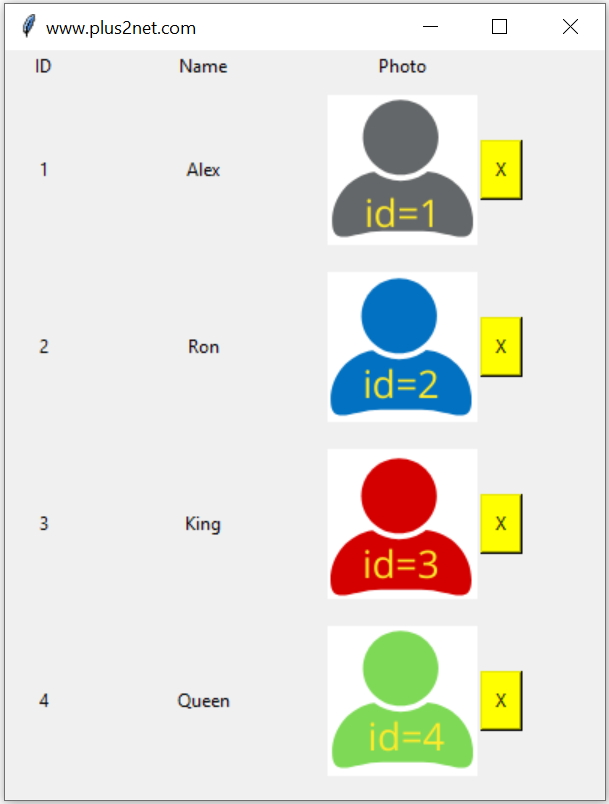# Delete Blob data column in Tkinter GUI

Python Tkinter MySQL Blob data type Display all imagesFor deleting record with blob data we will add delete function to our display record script. Here are the additional steps we will add to our display records script.

We will add one button to each row or record to trigger the delete operation.
1. The button once clicked will pass the record id to a function which will delete the particular record.
2. Show one confirmation message to the user asking to confirm the operation.
3. Based on confirmation or rejection, the delete operation should be executed.
4. After the delete operation we will refresh the view ( by deleting and adding all widgets ) so a fresh data is taken from backend MySQL database reflecting the changes by displaying the balance records.

Tkinter GUI application to add, display update and delete Blob column data with image of MySQL table

Read details of our student_profile table and how rows are displayed.

## Adding delete button to each record

The function display() is used to execute the SQL to collect the records and display data along with one delete button. Each time this function is called then the fresh data is displayed.
Once the delete button is pressed, it carries the student id as parameter to another function del_data(k). Check this code added to each delete button.
`command=lambda k=student: del_data(k)`

After deleting any record this function display() is called to show the records again.
``````def display(): # show all records
my_row=my_conn.execute("SELECT * FROM student_profile limit 0,4")
i=1 # data starts from row 1
global  images
for student in my_row:
stream = io.BytesIO(student)
img=Image.open(stream)
img = ImageTk.PhotoImage(img)
e = Label(my_w, text=student)
e = Label(my_w, text=student)
e = Label(my_w, image=img)
e = Button(my_w,bg='Yellow',
text='X',command=lambda k=student:del_data(k))
images.append(img) # garbage collection
i=i+1 ``````

## Delete record

The function del_data(s_id) is executed on click of the button ( as explained above ) and the student id ( s_id ) is received as parameter.
We will show one confirmation window asking user to confirm the operation. Read more on how to show message and collect the user choice here.
Based on the user confirmation the query to delete the row is executed. After deletion we removed all widgets and then call the function display() to refresh the data.
``````def del_data(s_id):
try:
"Are you sure ? ",icon='warning')
print(my_var)
if my_var:
query="DELETE FROM  student_profile WHERE id=%s"
my_data=[s_id]
my_conn.execute(query,my_data)
print("Row Deleted  ")

for row in my_w.grid_slaves():# remove widgets
row.grid_forget()
display() # refresh the list
except SQLAlchemyError as e:
error = str(e.__dict__['orig'])
print(error)``````
We defined my_conn as connection object Collect the data from student_profile table by using SQLALchemy.
Full code is here
``````# Deleting records with images
import tkinter  as tk
from tkinter import *
from sqlalchemy import create_engine
import io
from PIL import Image, ImageTk
from sqlalchemy.exc import SQLAlchemyError
from tkinter import messagebox as msg
my_w = tk.Tk() # parent window
my_w.geometry("400x500") # size as width height
# database connection
my_conn = create_engine("mysql+mysqldb://root:test@localhost/my_tutorial")
l1=Label(my_w, text='ID')
l1.grid(row=0,column=1)
l2=Label(my_w, text='Name')
l2.grid(row=0,column=2)
l3=Label(my_w, text='Photo')
l3.grid(row=0,column=3)
global img,images
images=[] # garbage collection
def display(): # show all records
my_row=my_conn.execute("SELECT * FROM student_profile limit 0,4")
i=1 # data starts from row 1
global  images
for student in my_row:
stream = io.BytesIO(student)
img=Image.open(stream)
img = ImageTk.PhotoImage(img)
e = Label(my_w, text=student)
e = Label(my_w, text=student)
e = Label(my_w, image=img)
e = Button(my_w,bg='Yellow',
text='X',command=lambda k=student:del_data(k))
images.append(img) # garbage collection
i=i+1

display()  # call to display all records
def del_data(s_id):
try:
"Are you sure ? ",icon='warning')
print(my_var)
if my_var:
query="DELETE FROM  student_profile WHERE id=%s"
my_data=[s_id]
my_conn.execute(query,my_data)
print("Row Deleted  ")

for row in my_w.grid_slaves():# remove widgets
row.grid_forget()
display() # refresh the list
except SQLAlchemyError as e:
error = str(e.__dict__['orig'])
print(error)
my_w.mainloop()``````

Subscribe to our YouTube Channel here

## Subscribe

* indicates required
Subscribe to plus2netplus2net.com